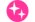Online ISSN : 2187-9761
ISSN-L : 2187-9761

この記事には本公開記事があります。本公開記事を参照してください。

ジャーナル フリー 早期公開

この記事には本公開記事があります。The final version of this article is now available: Vol. 86 (2020), No. 892 pp. 20-00102-20-00102

When a mobile robot moves in an environment where there are moving obstacles such as pedestrians and other robots, the robot is required to avoid collisions with these obstacles. However, trying to avoid all the predicted positions on the trajectory of the moving obstacle would overly restrict the robot’s movement. In order to solve this problem, we should be able to generate trajectories in which the robot and moving obstacles do not approach at the same time in the future. However, an approach such as optimizing the robot’s trajectory for the predicted trajectory of a moving obstacle would result in a very large number of states to be considered. This paper proposes a method to generate a set of candidate trajectories based on the robot’s motion model and determine the trajectories considering the collision probability with the predicted trajectories of moving obstacles at each time. In the proposed method, the action of the robot is determined by the following process. First, candidate trajectories of the robot based on its motion model are generated. Next, at each time step of each candidate trajectory, the collision probability with each obstacle is calculated. The same calculation is made for all obstacles and the collision probability with one or more obstacles is calculated. If the maximum probability of a candidate trajectory is greater than the threshold, the trajectory is considered dangerous and is removed from the candidate list. Depending on the distance from the target, the robot selects a trajectory from among the remaining candidate trajectories and determines the next action. In a simulation environment with 30 moving obstacles, the performance is compared with other methods and shown to be comparable or better. In addition, experiments using a real robot were conducted to show the usefulness of the proposed method.著者関連情報